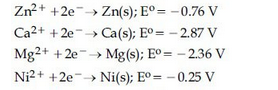Q

# How to solve this problem- Consider the following reduction processes The reducing power of the metals increases in order :

Consider the following reduction processesThe reducing power of the metals increases in order :

• Option 1)

Ca < Zn < Mg < Ni

• Option 2)

Ni < Zn < Mg < Ca

• Option 3)

Zn < Mg < Ni < Ca

• Option 4)

Ca < Mg < Zn < Ni

Views

Reduction -

Removal of Oxygen / electronegative element from a substance of addition of hydrogen / electropositive element to substance.

- wherein

e.g.

Electrode Potential -

The potential associated with each electrode is known as electrode potential.

-

Standard Electrode Potential Value --

Standard reduction potential -

It measure the tendency of a chemical species to acquire electrons and there by be reduced. It is measured in volts or mv.

-

Option 1)

Ca < Zn < Mg < Ni

Option 2)

Ni < Zn < Mg < Ca

Option 3)

Zn < Mg < Ni < Ca

Option 4)

Ca < Mg < Zn < Ni

Exams
Articles
Questions